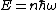# quantum acoustics

Also found in: Wikipedia.

## Quantum acoustics

The investigation of the effects of the laws of quantum mechanics on the propagation and absorption of sound. At the present stage of development of physical science, quantum mechanics is the most fundamental theory of physical phenomena. However, for many applications in the everyday world, a sufficiently accurate description of nature is provided by classical mechanics. Quantum acoustics refers to acoustic experiments that are carried out under conditions such that the results can be understood only in terms of quantum theory. As a general tendency, quantum effects become more important in acoustic experiments that are performed with higher-frequency sound waves or that are carried out at lower temperatures. See Quantum mechanics

#### Sound and phonons

To understand the quantum nature of sound, it is valuable to consider the origins of the quantum theory of light. Experiments in the latter part of the nineteenth century showed that the classical theory of electromagnetism combined with the laws of statistical mechanics could not explain the spectrum of light emitted by a heated surface. To reconcile theory and experiment, Max Planck in 1900 proposed that the energy of a light wave is quantized. This means that only certain values of the energy of the wave are allowed. These allowed values are given by ()where &planck; is Planck's constant divided by 2&pgr;, &ohgr; is the angular frequency of the light wave, and n is an integer. The modern interpretation of this formula describes this energy in terms of elementary quanta called photons. Each photon has an energy &planck;&ohgr;, and the wave is made up of n photons. It was later realized that this same quantization of energy should apply to sound waves. These fundamental units of sound energy were given the name phonons. See Heat radiation, Light, Phonon, Photon

#### Phonoatomic effect

One of the earliest experiments that confirmed the idea that light consists of photons was the photoelectric effect. In this effect the energy of light quanta is used to eject electrons from the surface of a metal. Only if the frequency of the light is sufficiently high do the photons have enough energy to knock the electrons out of the metal. The detection of this threshold frequency is thus strong evidence for quantization of energy. An analogous experiment has been performed with sound. In the experiment, sound is generated by a source in a vessel of liquid helium maintained at very low temperature, less than 0.1 K above absolute zero. In liquid helium, each atom is bound to the other atoms in the liquid by an energy that is unusually small compared to that of other liquids. For sound of sufficiently high frequency, the sound quanta when they arrive at the surface of the liquid have sufficient energy to knock helium atoms out of the liquid. These atoms can be detected by a suitable receiver placed above the surface of the liquid. The energy of an ejected atom, which determines its velocity, is equal to the energy of a phonon minus the binding energy. The time of arrival of the ejected atoms at the receiver is, in turn, determined by this velocity and hence is dependent on the frequency of the sound wave. Experiments have confirmed that the helium atoms arrive at the time expected based on this theory. See Photoemission

#### Phonon-phonon interactions

Sound waves in solids are attenuated during their propagation by a wide variety of physical processes. In many materials the most important mechanisms are related to impurities or defects in the solid, such as cracks, grain boundaries, or dislocations. Even when sound travels through a perfect crystal containing no defects, it is found that a measurable attenuation still occurs. In insulating crystals, where there are no free electrons, this attenuation is due to an interaction between the sound wave and the random thermal vibrations of the atoms in the solid. These random vibrations, which constitute the heat energy of the solid, are also quantized and are called thermal phonons. The attenuation of the sound wave can be attributed to collisions with the thermal phonons in which some of the sound quanta are scattered out of the sound beam. This mechanism is referred to as phonon-phonon scattering. See Crystal defects, Lattice vibrations, Sound absorption

In a linear elastic solid the elastic stress is exactly proportional to the strain, a phenomenon that is called Hooke's law. If Hooke's law holds, the presence of one wave does not affect the propagation of another, and so there are no interactions between phonons. Phonon-phonon scattering occurs because real solids always exhibit some deviations from linear elastic behavior. This nonlinearity is called anharmonicity. See Nonlinear acoustics

Even when the temperature is very low and there are very few thermal phonons, anharmonicity can still give rise to an attenuation of a sound wave. This is because the sound phonons can spontaneously decay into phonons of lower frequencies. The rate at which this decay occurs is proportional to the frequency of the sound wave to the fifth power, and so the attenuation is important only for sound waves of very high frequency. If the number of phonons is sufficiently large, it is possible under some circumstances for there to be a large buildup in the population of some of the decay phonons. This process is called parametric amplification.

## quantum acoustics

[′kwän·təm ə′kü·stiks]
(acoustics)
The study of the properties of propagating sound waves that are directly attributable to the underlying quantum-mechanical nature of the medium.
Site: Follow: Share:
Open / Close